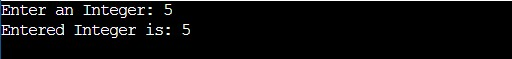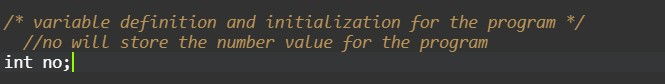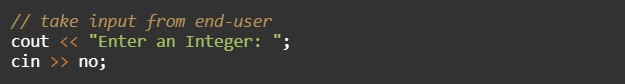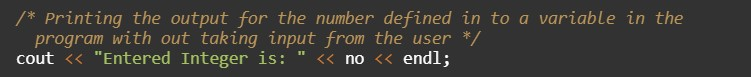# C++ Program to Print Number Entered by User

In this tutorial you will learn about the C++ Program to Print Number Entered by User and its application with practical example.

## C++ Program to Print Number Entered by User

In this tutorial, we will learn to create a C++ program that will Print Number Entered by Users in C++ programming.

## Prerequisites

Before starting with this tutorial we assume that you are best aware of the following C++ programming topics:

• Operators in C++ Programming.
• Basic Input and Output function in C++ Programming.
• Basic C++ Programming.

The printing of numbers from the user at a console is very essential. Number if can’t be printed on the screen then the user can never get the output for the calculation. That’s why number printing is important.

## Program to Print Number Entered by Users

In this program, we will take input numbers from the user and then we will print the number on the console with the help of a short c++ program.

With the help of this program, we can take input and Print Number Entered by Users.

## Output:-In the above program, we have first initialized the required variable.• no = it will hold the input number value from the user.

Taking Input from the user.Printing the data in the output in the number format.In this tutorial we have learn about the C++ Program to Print Number Entered by User and its application with practical example. I hope you will like this tutorial.